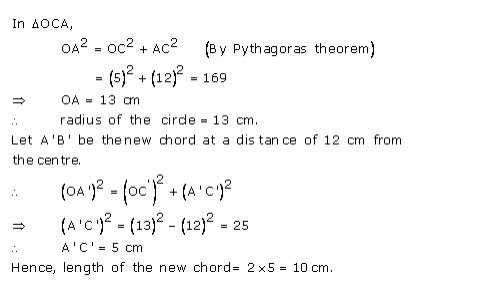# Selina Solutions Class 9 Mathematics Solutions for Exercise 17(A) in Chapter 17 - Chapter 17- Circles

A chord of length 24 cm is at a distance of 5 cm from the center of the circle. Find the length of the chord of the same circle which is at a distance of 12 cm from the center.

Let AB be the chord of length 24 cm and O be the center of the circle.

Let OC be the perpendicular drawn from O to AB.

We know, that the perpendicular to a chord, from the center of a circle, bisects the chord.

AC = CB = 12 cmVideo transcript
Related Questions

Lido

Courses

Teachers

Book a Demo with us

Syllabus

Maths
CBSE
Maths
ICSE
Science
CBSE

Science
ICSE
English
CBSE
English
ICSE
Coding

Terms & Policies

Selina Question Bank

Maths
Physics
Biology

Allied Question Bank

Chemistry
Connect with us on social media!